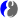# DMelt:Programming/5 Groovy

Limitted access. First login to DataMelt if you are a full DataMelt member. Then login to HandWiki as a user.

# Using Groovy

You can program in Groovy language as well. ReadGroovy (programming language). Groovy gets its attention because is designed to work seamlessly with the JVM, unlike Jython. One important advantage of Groovy scripting is that programs (especially loops) implemented in this language are 3-4 times faster than those in Jython, and much faster than in BeanShell.

Here is the Groovy tutorial Beginners Tutorial

You can use either Groovy Console (from the Run menu) or editor. In case of the editor, do not forget to use the extension ".groovy" ".gvy" or ".gy".

First, let us show how to make a standard Java JFrame:

```import javax.swing.JFrame
frame = new JFrame("Hello Swing")```

# Groovy and DataMelt

Let us show how to work with scientific libraries using Groovy.

Let us rewrite Jython/Python example of how to create a histogram and shown it using Groovy language.

First of all, one can use interactive Groovy shell of the IDE for (in the menu [Run]) or jut typing a code in the editor and saving it with the extension .gy or .groovy.

```import jhplot.HPlot
import jhplot.H1D

c1=new HPlot()
c1.setGTitle("Global labels: F_{2},  x_{&gamma;}  #bar{p}p F_{2}^{c#bar{c}}");
c1.visible()
c1.setAutoRange()

h1 = new H1D("Simple1",100, -2, 2.0)
rand =new java.util.Random()

print "Use of for loop in Groovy \n"
for (int i=0; i<100; i++) {
h1.fill(rand.nextGaussian())
}

c1.draw(h1)```

Create a file "example.gy" with this code (the extension ".gy" is very important!) and run this script as usual. To run this script, use the Run buttonon the toolbar of DataMelt. One can also use the [F8] key for fast execution of a script. In case of an error, the DataMelt outputs error to the Output console.

# Converting Jython example to Groovy examples

Since most of DataMelt example are written in Jython/Python, here are a few suggestions how to convert Jython code to Groovy scripts:

# Groovy and DataMelt libraries

Groovy scripts, if the run inside DataMelt, access to all numerical and scientific libraries of DataMelt. Here is one example: